# KSEEB Solutions for Class 8 Maths Chapter 11 Congruency of Triangles Ex 11.7

In this chapter, we provide KSEEB SSLC Class 8 Maths Chapter 11 Congruency of Triangles Ex 11.7 for English medium students, Which will very helpful for every student in their exams. Students can download the latest KSEEB SSLC Class 8 Maths Chapter 11 Congruency of Triangles Ex 11.7 pdf, free KSEEB SSLC Class 8 Maths Chapter 11 Congruency of Triangles Ex 11.7 pdf download. Now you will get step by step solution to each question.

### Karnataka State Syllabus Class 8 Maths Chapter 11 Congruency of Triangles Ex 11.7

Question 1.
In a triangle ABC, ∠B = 28° and ∠C= 56°. Find the largest and smallest side.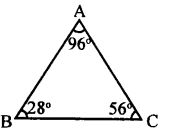∠A + ∠B + ∠C = 180°
[Sum of the angles of a triangle]
∠A + 28 + 56 = 180°
∠A + 84° = 180°
∠A = 180 – 84
∠A = 96°
BC is the largest side
[Opposite to largest angle ∠A]
AC is the smallest side
[Opposite to smalles angle ∠B]

Question 2.
In a triangle ABC, we have AB = 4cm, BC = 5.6 cm and CA = 7.6 cm. Write the angles of the triangle in ascending order
of measures.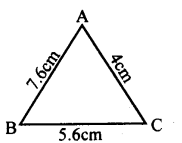∠C <∠A <∠B

Question 3.
Let ΔABC be a triangle such that ∠B = 70° and∠C = 40°. suppose D is a point on BC such that AB = AD. Prove that AB > CD.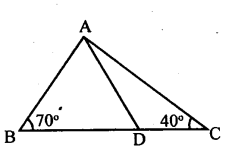In Δ ABD, AB = AD
∴ ∠ABD = ∠ APB = 70° [Theorem]
∠APB +∠ ADC = 180° [Linear pair]
∠DAC = 180 – ( 110 + 40)
= 180 – 150
∠DAC = 30°
∠ACD > ∠DAC
∴ AB > CD

Question 4.
Let ABCD be a quadrilateral in which AD is the largest side and BC is the smallest side. Prove that|A <[C. [Hint: Join AC]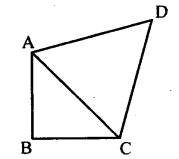Join AC
In Δ ABC   AB > BC [BC is the smallest side]
∴ ∠ACB > ∠BAC …(i)
∠ACD > ∠PAC …(ii)
∠ACB + ∠ACD > ∠BAC + ∠DAC
∴∠A < ∠C

Question 5.
Let ABC be a triangle and P be an interior point, prove that AB + BC + CA < 2(PA + PB + PC).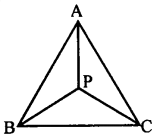In ΔPBA, AB < PA + PB ….(i)
In ΔPBC, BC < PB + PC ….(ii)
In ΔPCA, AC < PC + PA ….(iii)
By adding (i), (ii), and (iii)
AB + BC + AC < PA + PB + PB + PC + PC + PA > 2PA + 2PB + 2PC
AB + BC + AC < 2 (PA + PB + PC).

All Chapter KSEEB Solutions For Class 8 maths

—————————————————————————–

All Subject KSEEB Solutions For Class 8

*************************************************

I think you got complete solutions for this chapter. If You have any queries regarding this chapter, please comment on the below section our subject teacher will answer you. We tried our best to give complete solutions so you got good marks in your exam.

If these solutions have helped you, you can also share kseebsolutionsfor.com to your friends.

Best of Luck!!